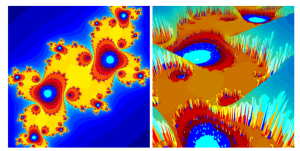## PDEs and iGem

One of the first equations I discretized and simulated was the wave equation using a finite difference scheme. I ended up with a simulation, which is quite pretty:

The biggest PDE project I have done so far was with the Leuven iGem-Team. We simulated the behavior of pattern forming bacteria using pure PDE and a PDE-Agent hybrid models. The pure PDE simulations have been generated by discretizing a modified Keller-Segel system of equations using a finite volume method in Matlab:

In order to take cell adhesion into account we created this hybrid model:

The international jury in Boston nominated us for the best model award, which means they saw us in the top 5 of overgrad teams in the modeling category.
Leuven iGem 2015 wiki

## Ray Tracing

Ray tracing is a fascinating algorithm used for image synthesis. I have written a rudimentary ray tracer consisting of more then 5000 lines of code.
The video below shows it render a high resolution triangle mesh representation of the Stanford dragon:

Fast implementations depend on binary trees to reduce the number of required ray triangle intersection computations. These trees are quite beautiful to look at. In a nutshell such trees are generated by splitting a bounding box which contains the object in two recursively again and again. The video below visualizes such a tree:

Please note how the outermost box shows up for high sensitivity values and the splitted sub boxes become visible as the sensitivity is reduced. When it is reduced further, the edges of the dragon become visible nicely.

The same algorithm can also be used for mathematical plotting purposes. Below you can see renderings of a Julia fractal in two and three dimensions, generated using the same ray tracing code: